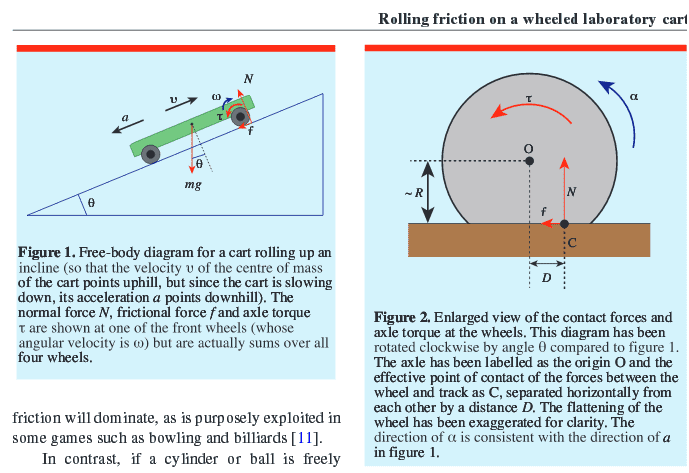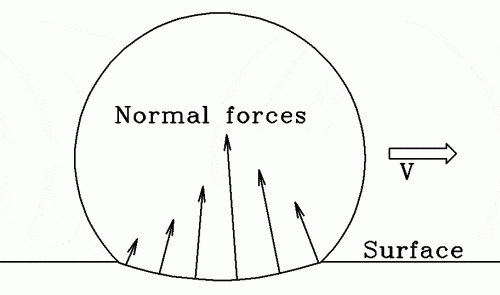# Coefficient of rolling friction for a lab cart

• I
Einstein44
I was looking for a way to calculate the friction arising from the axle and wheel of a standard lab cart. I came across this research paper: https://www.usna.edu/Users/physics/mungan/_files/documents/Publications/PhysEd4.pdf
That derived the following equation for the coefficient of rolling friction:
$$\mu _{r}\approx \frac{D+kr}{R}$$
for what the variables stand can be seen on the diagram shown in the article (it would be too difficult to explain this with words).
Now my problem is that I had never heard of a such thing and want able to find much on the internet that explained it in detail. I wasn't able to follow the derivation exactly, but I think some people here will find it easier to follow.
My question is: is this really a correct indicator of the friction for a cart moving down a ramp, and how can this be used to find the frictional Force? Can you just simply use the same approach as for dynamic friction for instance? (just multiplying it by the Normal Force?)Gold Member
The first part ##D /R## is usually referred to as rolling resistance. By definition (sum of moments):
$$f R = ND$$
or:
$$\frac{D}{R} = \frac{f}{N}= C_{rr}$$
Where ##C_{rr}## is the coefficient of rolling resistance. So there is no friction, but since the resistance force has a definition similar to a friction force, they are used in a similar way.

The second part ##k r/R## is the friction force from the fixed axle-wheel contact patch ##f_a = kN## as felt at wheel-road contact patch, ##f_w##. Again sum of moments:
$$f_a r = f_w R$$
or:
$$f_w = \frac{f_a r}{R}= \frac{k r}{R}N$$
$$\mu_r N = f + f_w = C_{rr}N + \frac{k r}{R}N = \frac{D}{R}N + \frac{k r}{R}N = \frac{D+k r}{R}N$$
$$\mu_r = \frac{D+k r}{R}$$

•Einstein44 and Lnewqban
Homework Helper
Gold Member
@jack action, why is N off center and what distance D depend on?

@Einstein44, consider that the values of N will be different for front and rear wheels, due to the slope.

Einstein44
The first part ##D /R## is usually referred to as rolling resistance. By definition (sum of moments):
$$f R = ND$$
or:
$$\frac{D}{R} = \frac{f}{N}= C_{rr}$$
Where ##C_{rr}## is the coefficient of rolling resistance. So there is no friction, but since the resistance force has a definition similar to a friction force, they are used in a similar way.

The second part ##k r/R## is the friction force from the fixed axle-wheel contact patch ##f_a = kN## as felt at wheel-road contact patch, ##f_w##. Again sum of moments:
$$f_a r = f_w R$$
or:
$$f_w = \frac{f_a r}{R}= \frac{k r}{R}N$$
$$\mu_r N = f + f_w = C_{rr}N + \frac{k r}{R}N = \frac{D}{R}N + \frac{k r}{R}N = \frac{D+k r}{R}N$$
$$\mu_r = \frac{D+k r}{R}$$
Thank you! On the website you have linked in your comment above I have found the following equation:
$$F=\frac{Nb}{r}$$
Would this then give me the force due to the rolling resistance directly? That means I wouldn't need to use the equation above? Because essentially I am trying to find out the Frictional Force.

Gold Member
@jack action, why is N off center and what distance D depend on?
https://en.wikipedia.org/wiki/Rolling_resistance said:
It is mainly caused by non-elastic effects; that is, not all the energy needed for deformation (or movement) of the wheel, roadbed, etc., is recovered when the pressure is removed. Two forms of this are hysteresis losses (see below), and permanent (plastic) deformation of the object or the surface (e.g. soil).

Would this then give me the force due to the rolling resistance directly?
Normally, you used ##F = C_{rr} N## as the values of rolling resistance coefficients are usually easy to find. I never saw a source for ##b## except for the one I cited (and there are not a lot except for steel-on-steel).

The equation from the OP is usually used by more advanced designs like bearing manufacturing for research purposes.

•Einstein44 and Lnewqban
Homework Helper
Gold Member
Einstein44
Normally, you used ##F = C_{rr} N## as the values of rolling resistance coefficients are usually easy to find. I never saw a source for ##b## except for the one I cited (and there are not a lot except for steel-on-steel).

The equation from the OP is usually used by more advanced designs like bearing manufacturing for research purposes.
Yes, I understand what you wrote. However, I feel like this mainly applies to wheels that deform on the surface, such as a deflated tire for example (correct me if I'm wrong), so how can you apply any equation to find the Frictional Force for basically a hard wheel on another surface? (besides approximating D from the first equation to be zero, due to no deformation of the wheel)

••